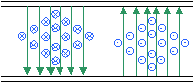;Please Support RF Cafe by purchasing my  ridiculously low−priced products, all of which I created.

RF Workbench  (shareware)# Rectangular & Circular Waveguide: Equations, Fields, & fco Calculator

The following equations and images describe electromagnetic waves inside both rectangular waveguide and circular (round) waveguides. Oval waveguide equations are not included due to the mathematical complexity.

A Cavity Resonance Calculator is included in Espresso Engineering Workbook™ for FREE.

Rectangular Waveguide Cutoff Frequency

The lower cutoff frequency (or wavelength) for a particular mode in rectangular waveguide is determined by the following equations (note that the length, x, has no bearing on the cutoff frequency):Cutoff Frequency Calculator

This example is for TE1,0 (the mode with the lowest cutoff frequency) in WR284 waveguide (commonly used for S-band radar systems). It has a width of 2.840" (7.214 cm) and a height of 1.340"(3.404 cm).

 where a =b =m =n =ε =µ = Inside width (m), longest dimensionInside height (m), shortest dimensionNumber of ½-wavelength variations of fields in the "a" directionNumber of ½-wavelength variations of fields in the "b" direction Permittivity (8.854187817E-12 for free space)Permeability (4πE-7 for free space)

TE (Transverse Electric) Mode

The TE10 mode is the dominant mode of a rectangular waveguide with a>b, since it has the lowest attenuation of all modes. Either m or n can be zero, but not both.End View (TE10)Side View (TE10)Top View (TE10)

____ Electric field lines

p    _ _ _ Magnetic field lines

TM (Transverse Magnetic) Mode

For TM modes, m=0 and n=0 are not possible, thus, TM11 is the lowest possible TM mode.End View (TM11)Side View (TM11)

____ Electric field lines

_ _ _ Magnetic field lines

TE (Transverse Electric) ModeThe lower cutoff frequency (or wavelength) for a particular TE mode in circular waveguide is determined by the following equation:, where p'mn is

 m p'm1 p'm2 p'm3 0 3.832 7.016 10.174 1 1.841 5.331 8.536 2 3.054 6.706 9.970

TM (Transverse Magnetic) Mode

The lower cutoff frequency (or wavelength) for a particular TM mode in circular waveguide is determined by the following equation:(m), where pmn is

 m pm1 pm2 pm3 0 2.405 5.520 8.654 1 3.832 7.016 10.174 2 5.135 8.417 11.620

Related Pages on RF Cafe